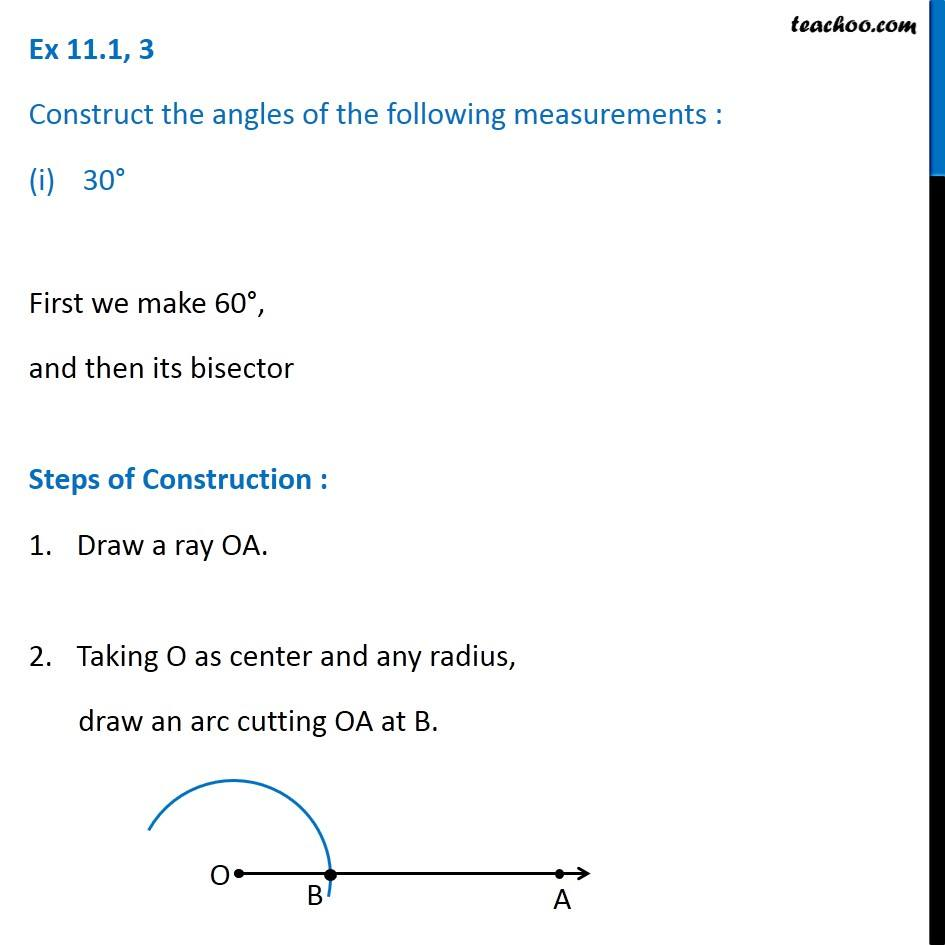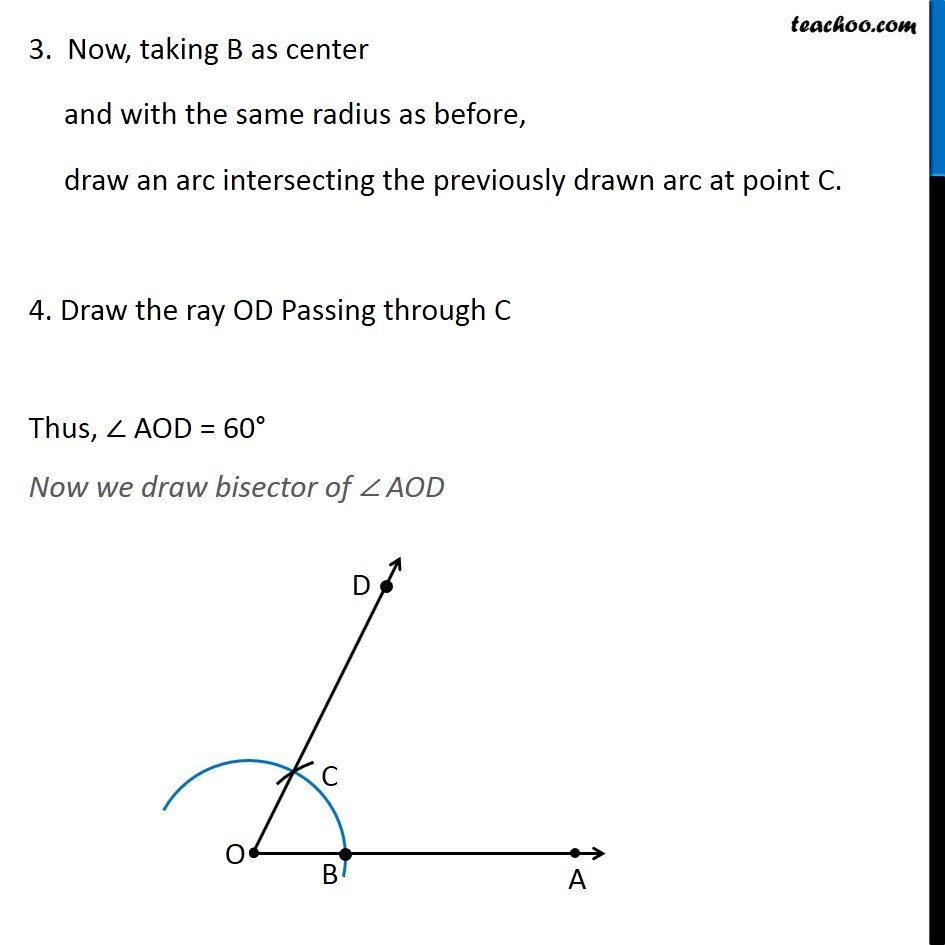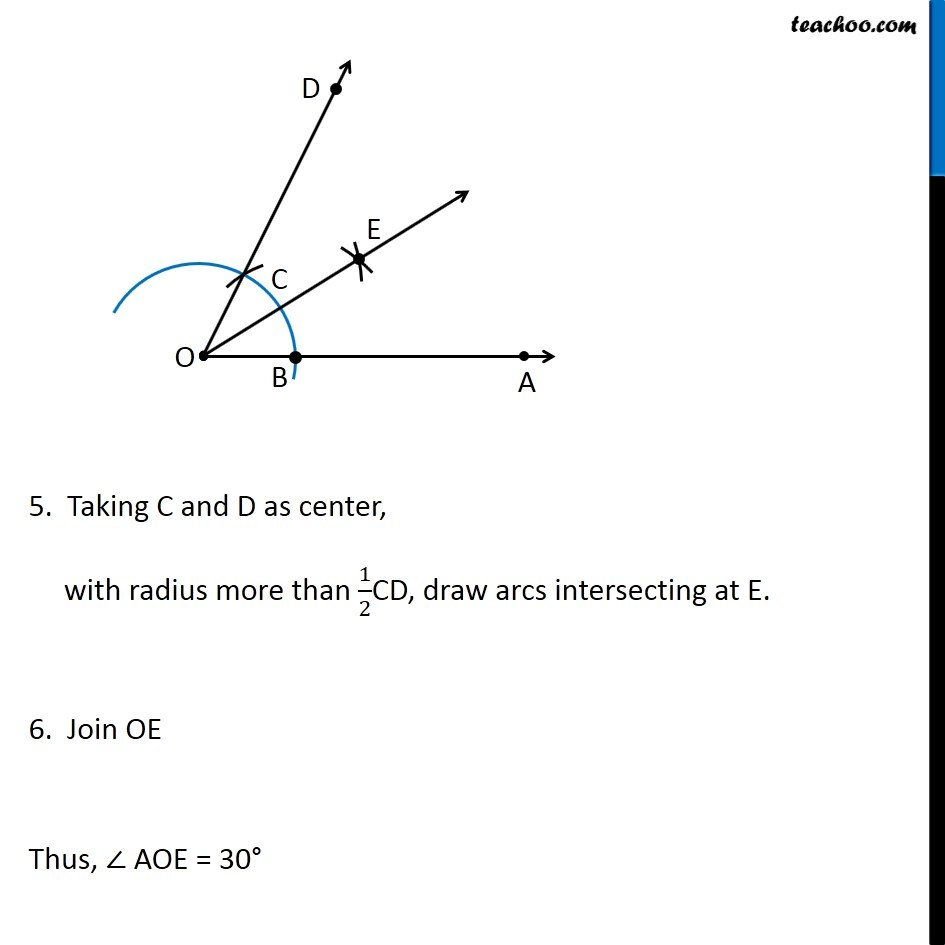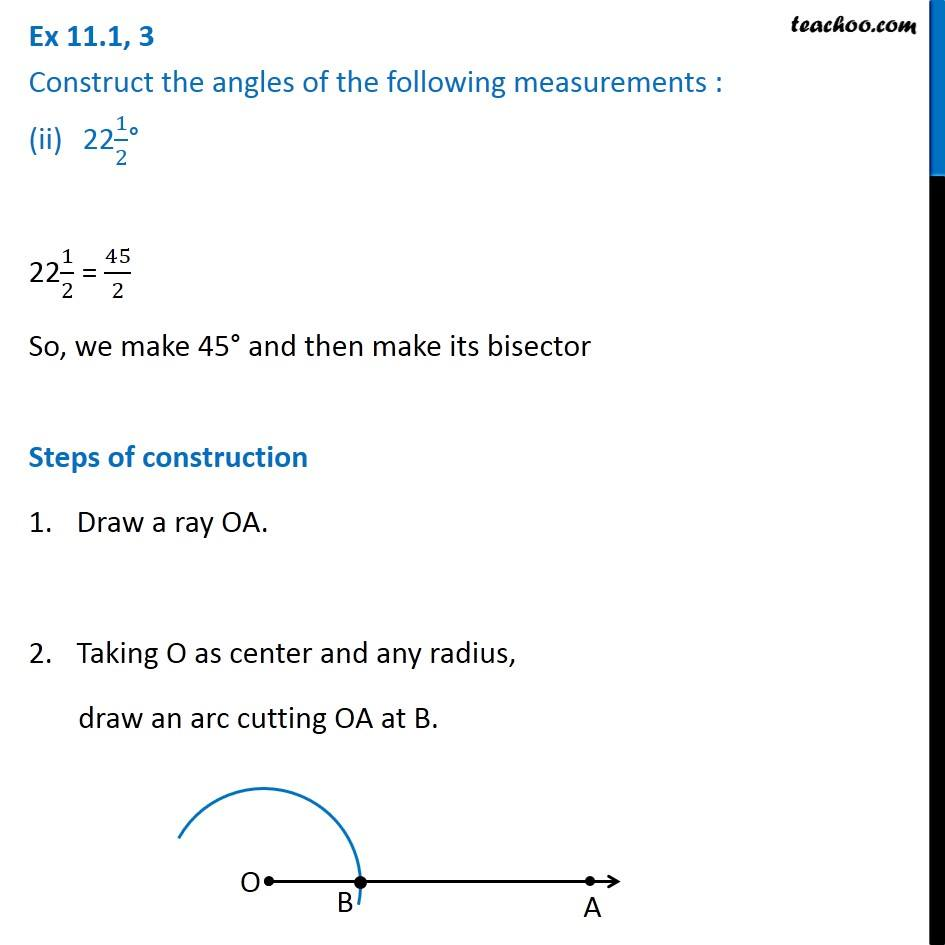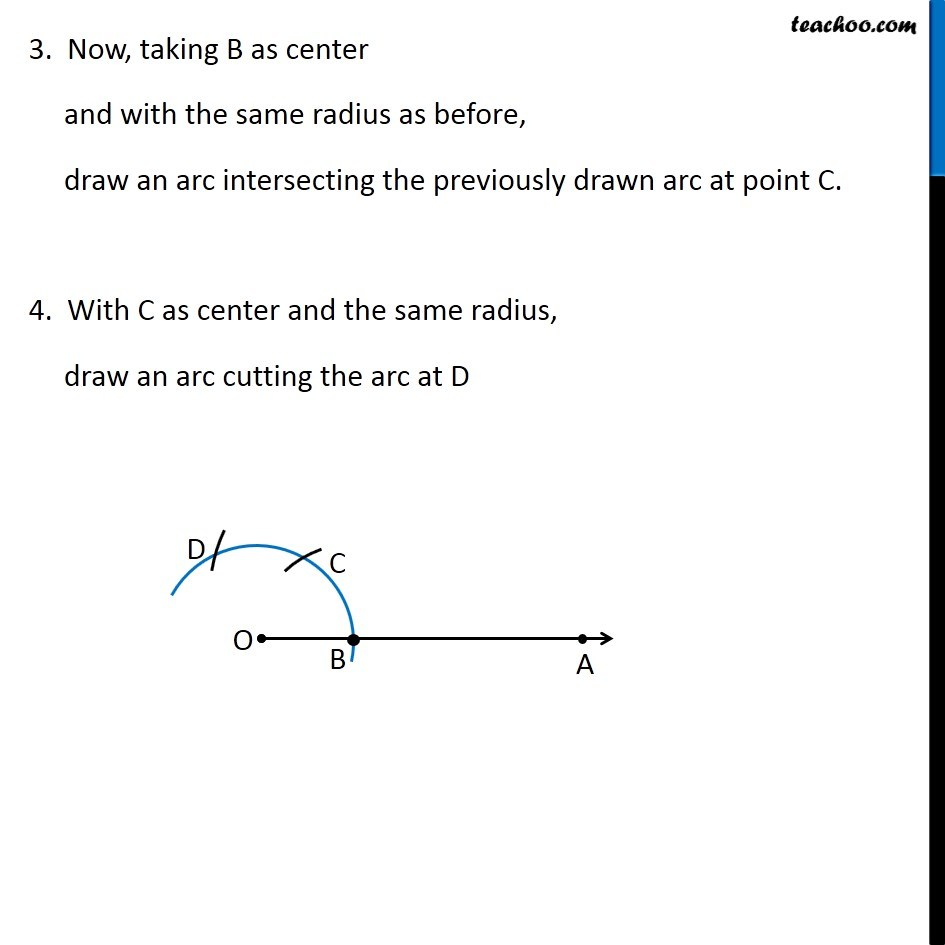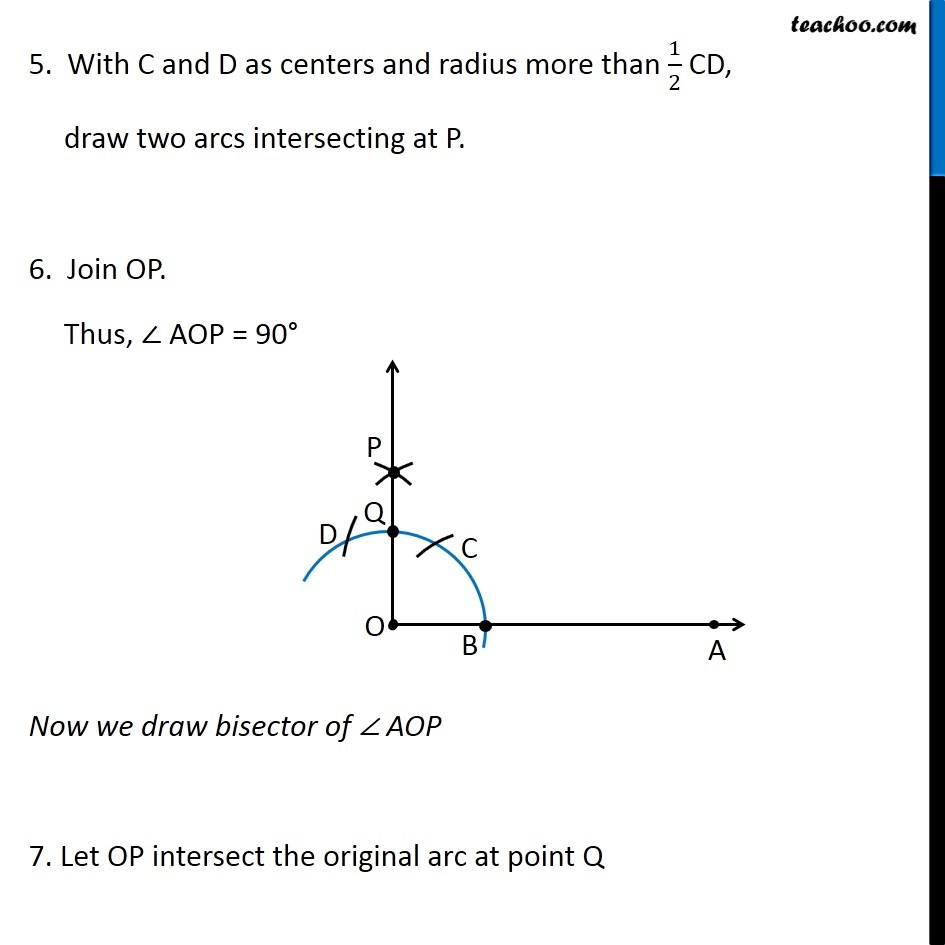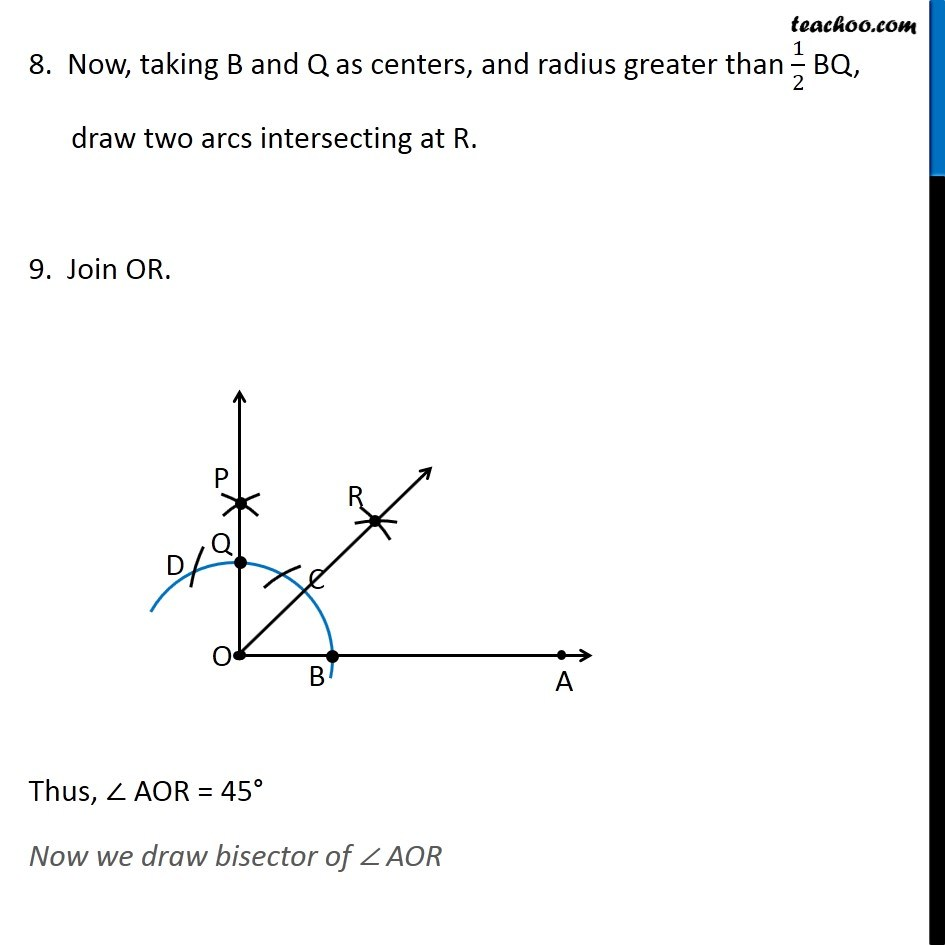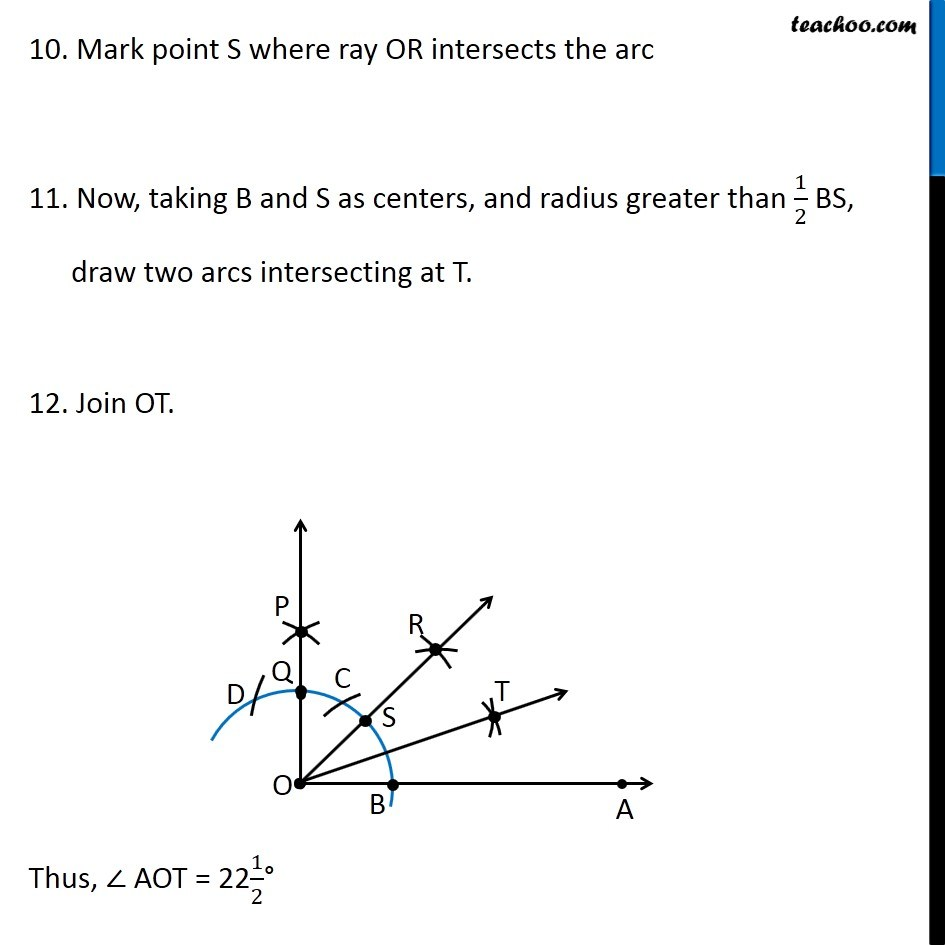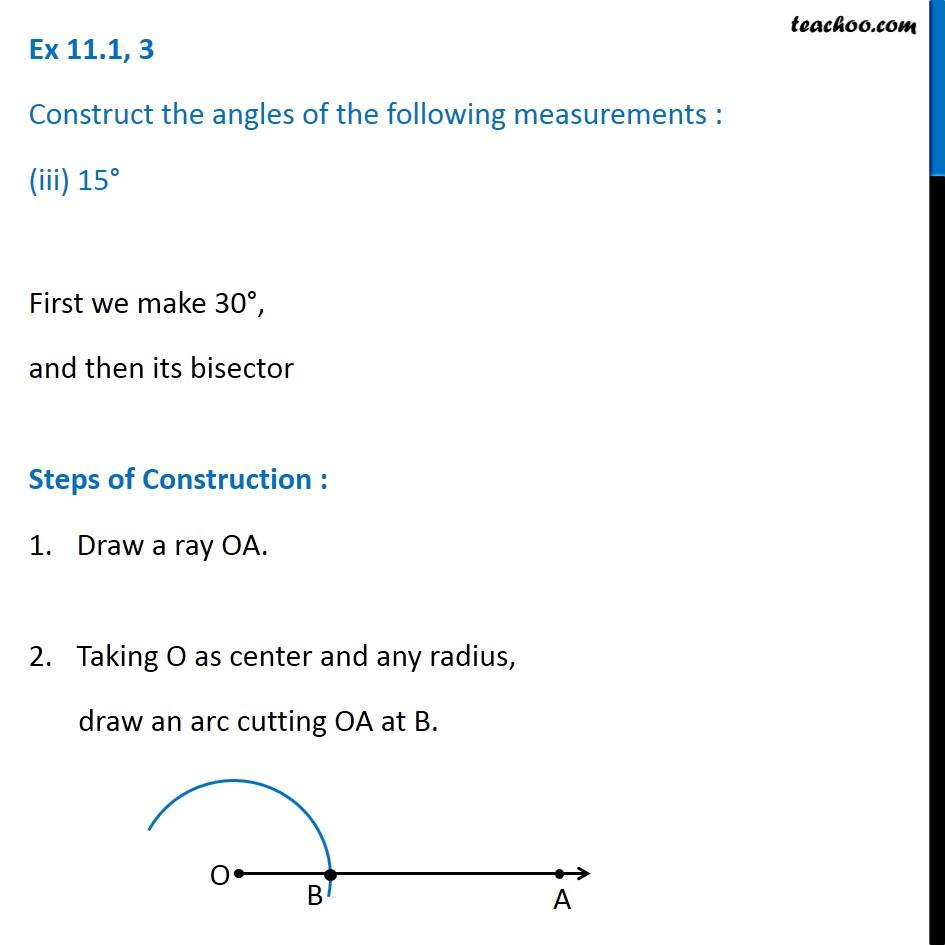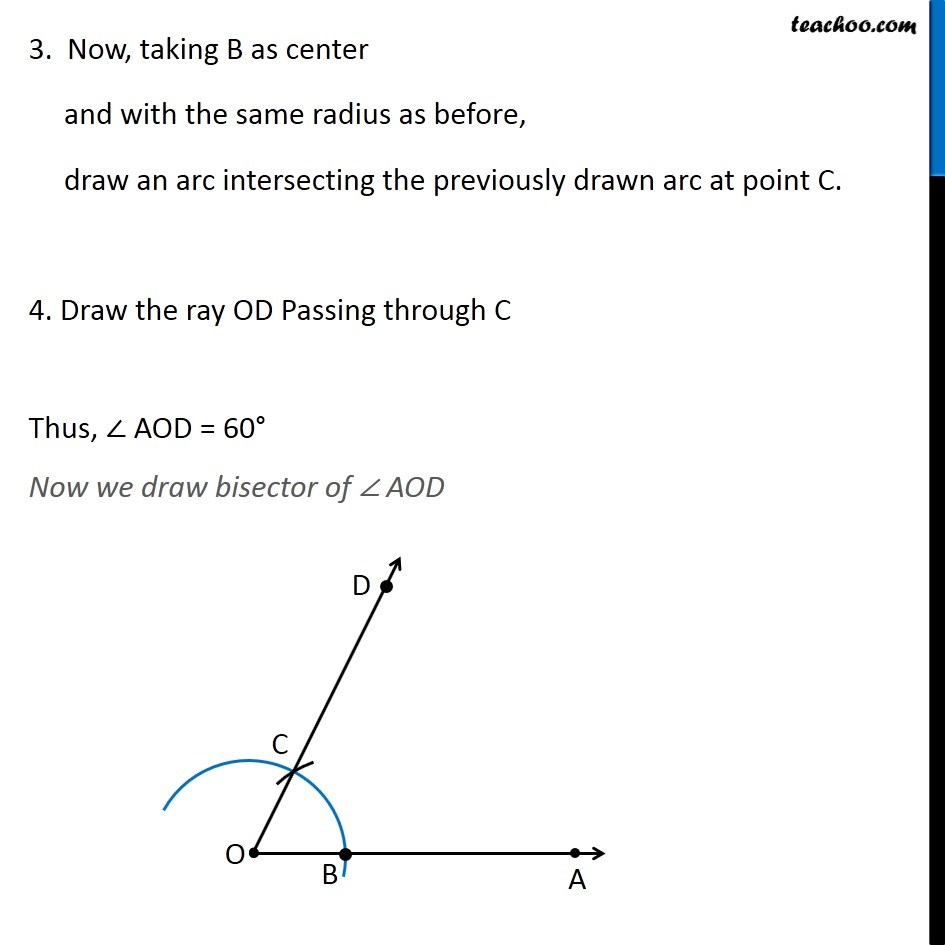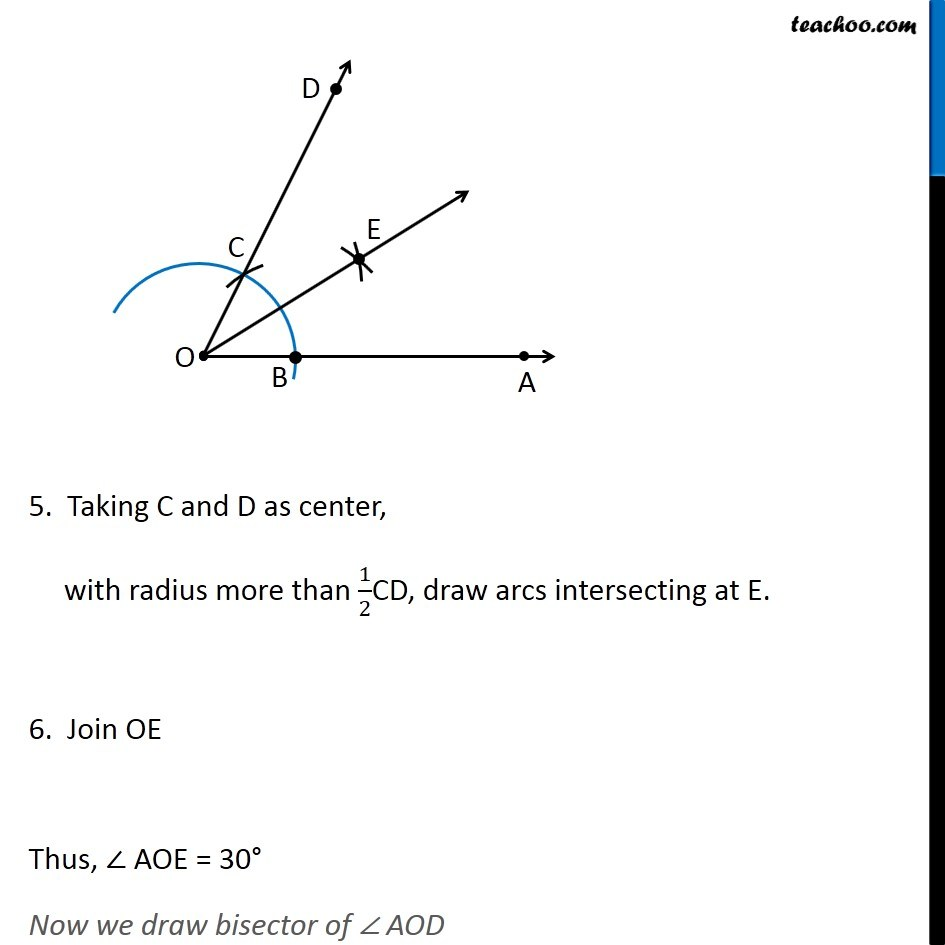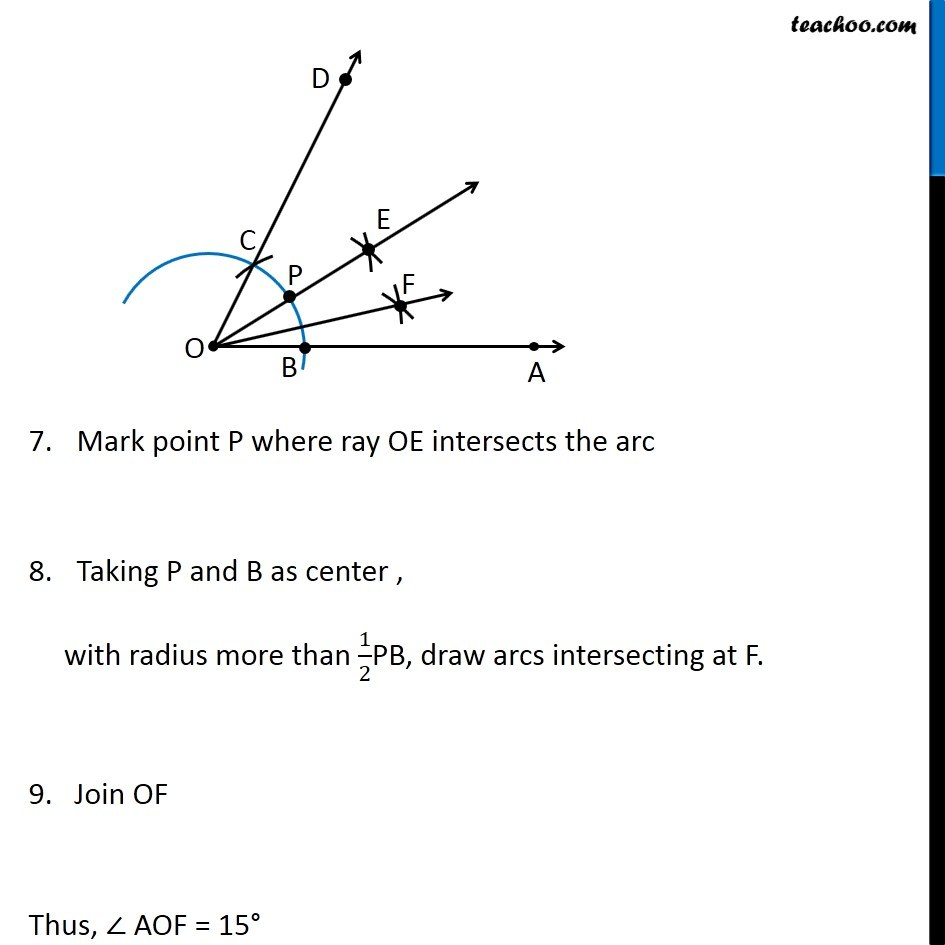1. Chapter 11 Class 9 Constructions
2. Serial order wise
3. Ex 11.1

Transcript

Ex 11.1, 3 Construct the angles of the following measurements : 30° First we make 60°, and then its bisector Steps of Construction : Draw a ray OA. Taking O as center and any radius, draw an arc cutting OA at B. 3. Now, taking B as center and with the same radius as before, draw an arc intersecting the previously drawn arc at point C. 4. Draw the ray OD Passing through C Thus, ∠ AOD = 60° Now we draw bisector of ∠ AOD . 5. Taking C and D as center, with radius more than 1/2CD, draw arcs intersecting at E. 6. Join OE Thus, ∠ AOE = 30° Ex 11.1, 3 Construct the angles of the following measurements : 221/2° 221/2 = 45/2 So, we make 45° and then make its bisector Steps of construction Draw a ray OA. Taking O as center and any radius, draw an arc cutting OA at B. 3. Now, taking B as center and with the same radius as before, draw an arc intersecting the previously drawn arc at point C. 4. With C as center and the same radius, draw an arc cutting the arc at D 5. With C and D as centers and radius more than 1/2 CD, draw two arcs intersecting at P. 6. Join OP. Thus, ∠ AOP = 90° Now we draw bisector of ∠ AOP 7. Let OP intersect the original arc at point Q 8. Now, taking B and Q as centers, and radius greater than 1/2 BQ, draw two arcs intersecting at R. 9. Join OR. Thus, ∠ AOR = 45° Now we draw bisector of ∠ AOR 10. Mark point S where ray OR intersects the arc 11. Now, taking B and S as centers, and radius greater than 1/2 BS, draw two arcs intersecting at T. 12. Join OT. Thus, ∠ AOT = 221/2° Ex 11.1, 3 Construct the angles of the following measurements : (iii) 15° First we make 30°, and then its bisector Steps of Construction : Draw a ray OA. Taking O as center and any radius, draw an arc cutting OA at B. 3. Now, taking B as center and with the same radius as before, draw an arc intersecting the previously drawn arc at point C. 4. Draw the ray OD Passing through C Thus, ∠ AOD = 60° Now we draw bisector of ∠ AOD 5. Taking C and D as center, with radius more than 1/2CD, draw arcs intersecting at E. 6. Join OE Thus, ∠ AOE = 30° Now we draw bisector of ∠ AOD Mark point P where ray OE intersects the arc Taking P and B as center , with radius more than 1/2PB, draw arcs intersecting at F. 9. Join OF Thus, ∠ AOF = 15°

Ex 11.1

About the AuthorDavneet Singh
Davneet Singh is a graduate from Indian Institute of Technology, Kanpur. He has been teaching from the past 9 years. He provides courses for Maths and Science at Teachoo.